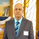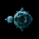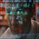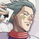7206 görüntülenme
7206
This indicator displays the trend-adjusted price activity of a security. It oscillates around the zero-line. It is usually plotted as OHLC, but Pine doesn't have this support yet. So, I have rendered OHLC separately (see chart for the details).

This indicator is particularly useful for ‘scalping’ in a sideways market, where there is limited movement taking place, rather than a trending market undergoing larger swing movements.

I am not sure how much this resembles the commercial indicator out there as I don't have access to one. This is a direct port of a similar TOS indicator.

```//
// @author LazyBear
//
// If you use this code in its original/modified form, do drop me a note.
//
study("ValueChart Indicator [LazyBear]", shorttitle="ValueChart_LB")

prev(s,i) =>
y=abs(round(i))
s[y]

length = input(5, title="Number of bars")
extTop = input(12, title="Extreme Level Top")
extBot = input(-12, title="Extreme Level Bottom")
sigTop = input(8, title="Significant Level Top")
sigBot = input(-8, title="Significant Level Bottom")
fairTop = input(4, title="Fair Value Top")
fairBot = input(-4, title="Fair Value Bottom")

ext_l = plot(extTop,title="Extreme Top Line",color=red)
exb_l = plot(extBot,title="Extreme Bottom Line",color=red)
top_l=plot(sigTop,title="Top Chart Line",color=red, style=3)
bot_l=plot(sigBot, title="Bottom Chart Line",color=red, style=3)
tm_l=plot(fairTop,title="Top Mid Chart Line",color=gray)
bm_l=plot(fairBot,title="Bottom Mid Chart Line",color=gray)
fill(ext_l, top_l, red)
fill(exb_l, bot_l, red)
fill(top_l, tm_l, yellow, transp=80)
fill(bot_l, bm_l, yellow, transp=80)
fill(tm_l, bm_l, green, transp=85)

varp = round(length/5)
h_f = length > 7

vara=h_f ? highest(high,varp)-lowest(low,varp) : 0
varr1 = h_f ? iff(vara==0 and varp==1,abs(close-prev(close,-varp)),vara) : 0
varb=h_f ? prev(highest(high,varp),-varp+1)-prev(lowest(low,varp),-varp) : 0
varr2 = h_f ? iff(varb==0 and varp==1,abs( prev(close,-varp)-prev(close,-varp*2) ),varb) : 0
varc=h_f ? prev(highest(high,varp),-varp*2)-prev(lowest(low,varp),-varp*2) : 0
varr3 = h_f ? iff(varc == 0 and varp==1,abs(prev(close,-varp*2)-prev(close,-varp*3)),varc) : 0
vard = h_f ? prev(highest(high,varp),-varp*3)-prev(lowest(low,varp),-varp*3) : 0
varr4 = h_f ? iff(vard == 0 and varp==1,abs(prev(close,-varp*3)-prev(close,-varp*4)),vard)  : 0
vare = h_f ? prev(highest(high,varp),-varp*4)-prev(lowest(low,varp),-varp*4) : 0
varr5 = h_f ? iff(vare == 0 and varp==1,abs(prev(close,-varp*4)-prev(close,-varp*5)),vare) : 0
cdelta = abs(close - prev(close,-1))
var0 = (not h_f) ? iff((cdelta > (high-low)) or (high==low),cdelta,(high-low)) : 0
lrange=h_f ? ((varr1+varr2+varr3+varr4+varr5)/5)*.2 : sma(var0,5)*.2

mba = sma( (high+low)/2,length)
vopen = (open- mba)/lrange
vhigh = (high-mba)/lrange
vlow = (low-mba)/lrange
vclose = (close-mba)/lrange

plot(vhigh, color=maroon, linewidth=1)
plot(vopen, linewidth=2, color=green)
plot(vclose, linewidth=2, color=vclose > vopen ? blue : red, style=3)
plot(vlow, color=maroon, linewidth=1)
```prev(s,i) =>
y=abs(round(i))
s
What are the values for i and s? Thanks.
Cevap GönderLazy Bear, this is a great tool to use when paired up with Pivot Points! Confirms/Denies probability of price pushing through a pivot or not.
Thanks!
Cevap GönderGood job, what is the time frame for this value chart?
Cevap GönderLEONES
@TheLark found M15 effective (see his comment above).
Cevap GönderLazyBear
yes this is the Mark Heldweg indicator
Cevap GönderLazyBear
I think it is based on a 5day average
Cevap Gönderthe original recalculates and has about 70% correct signals, its the same here ?
Cevap Gönderglaz
AFAIK, "recalculate" (or repaint) shouldn't happen. I will let somebody who uses this indicator regularly to comment on the "70% correct" query.
Cevap Gönder